If there is one prayer that you should pray/sing every day and every hour, it is the LORD's prayer (Our FATHER in Heaven prayer)
It is the most powerful prayer. A pure heart, a clean mind, and a clear conscience is necessary for it.
- Samuel Dominic Chukwuemeka

For in GOD we live, and move, and have our being. - Acts 17:28

The Joy of a Teacher is the Success of his Students. - Samuel Chukwuemeka

# Solved Examples on Trigonometry (All Topics)Verify your answers with these Calculators as applicable.
For ACT Students
The ACT is a timed exam...$60$ questions for $60$ minutes
This implies that you have to solve each question in one minute.
Some questions will typically take less than a minute a solve.
Some questions will typically take more than a minute to solve.
The goal is to maximize your time. You use the time saved on those questions you solved in less than a minute, to solve the questions that will take more than a minute.
So, you should try to solve each question correctly and timely.
So, it is not just solving a question correctly, but solving it correctly on time.
Please ensure you attempt all ACT questions.
There is no "negative" penalty for any wrong answer.

For JAMB Students
Calculators are not allowed. So, the questions are solved in a way that does not require a calculator.

For WASSCE Students
Any question labeled WASCCE is a question for the WASCCE General Mathematics
Any question labeled WASSCE:FM is a question for the WASSCE Further Mathematics/Elective Mathematics

For GCSE and Malta Students
All work is shown to satisfy (and actually exceed) the minimum for awarding method marks.
Calculators are allowed for some questions. Calculators are not allowed for some questions.

For NSC Students
For the Questions:
Any space included in a number indicates a comma used to separate digits...separating multiples of three digits from behind.
Any comma included in a number indicates a decimal point.
For the Solutions:
Decimals are used appropriately rather than commas
Commas are used to separate digits appropriately.

Solve all questions.
Draw diagrams as applicable.
Show all work.

NSC (1.1) Without using a calculator, write the following expressions in terms of $\sin 11^\circ$:
(1.1.1) $\sin 191^\circ$
(1.1.2) $\cos 22^\circ$

(1.2) Simplify $\cos (x - 180^\circ) + \sqrt{2}\sin (x + 45^\circ)$ to a single trigonometric ratio.

(1.3) Given: $\sin P + \sin Q = \dfrac{7}{5}$ and $\hat{P} + \hat{Q} = 90^\circ$

Without using a calculator, determine the value of $\sin 2P$

$(1.1.1) \\[3ex] \sin 191 \\[3ex] = \sin(180 + 11)...Sum\;\;Formula \\[3ex] = \sin 180 \cos 11 + \cos 180 \sin 11 \\[3ex] = 0 * \cos 11 + -1 * \sin 11 \\[3ex] = 0 + -\sin 11 \\[3ex] = 0 - \sin 11 \\[3ex] = -\sin 11 \\[3ex] (1.1.2) \\[3ex] \cos 22 \\[3ex] = \cos (2 * 11)...Double-Angle\;\;Formula \\[3ex] = 1 - 2\sin^2(11) \\[3ex] (1.2) \\[3ex] \cos (x - 180^\circ) + \sqrt{2}\sin (x + 45^\circ) \\[3ex] \cos (x - 180) \\[3ex] = \cos x \cos 180 + \sin x \sin 180 ...Difference\;\;Formula \\[3ex] = \cos x * -1 + \sin x * 0 \\[3ex] = -\cos x + 0 \\[3ex] = -\cos x \\[3ex] \sin(x + 45) \\[3ex] = \sin x \cos 45 + \cos x \sin 45 ...Sum\;\;Formula \\[3ex] = \sin x \left(\dfrac{\sqrt{2}}{2}\right) + \cos x\left(\dfrac{\sqrt{2}}{2}\right) \\[5ex] = \dfrac{\sqrt{2}}{2} \sin x + \dfrac{\sqrt{2}}{2} \cos x \\[5ex] \sqrt{2} \sin (x + 45) \\[3ex] = \sqrt{2}\left(\dfrac{\sqrt{2}}{2} \sin x + \dfrac{\sqrt{2}}{2} \cos x\right) \\[5ex] = \sin x + \cos x \\[3ex] \implies \\[3ex] \cos (x - 180^\circ) + \sqrt{2}\sin (x + 45^\circ) \\[3ex] = -\cos x + \sin x + \cos x \\[3ex] = \sin x \\[3ex] (5.3) \\[3ex] \hat{P} + \hat{Q} = 90 \implies \hat{P} \;\;and\;\; \hat{Q} \;\;are\;\;complements \\[3ex] \implies \sin Q = \cos P ...cofunctions\;\;of\;\;complementary\;\;\angle s \\[3ex] \sin P + \sin Q = \dfrac{7}{5} \\[5ex] \implies \sin P + \cos P = \dfrac{7}{5} \\[5ex] Square\;\;both\;\;sides \\[3ex] (\sin P + \cos P)^2 = \left(\dfrac{7}{5}\right)^2 \\[5ex] (\sin P + \cos P)(\sin P + \cos P) = \dfrac{49}{25} \\[5ex] \sin^2P + \sin P \cos P + \cos P \sin P + \cos^2P = \dfrac{49}{25} \\[5ex] \sin^2P + 2\sin P \cos P + \cos^2P = \dfrac{49}{25} \\[5ex] \sin^2P + \cos^2P + 2\sin P \cos P = \dfrac{49}{25} \\[5ex] \sin^2P + \cos^2P = 1 ...Pythagorean\;\;Identity \\[3ex] \implies 1 + 2\sin P \cos P = \dfrac{49}{25} \\[5ex] 2\sin P \cos P = \dfrac{49}{25} - 1 \\[5ex] 2\sin P \cos P = \dfrac{49}{25} - \dfrac{25}{25} \\[5ex] 2\sin P \cos P = \dfrac{24}{25}$
(2.) JAMB A chord of a circle subtends an angle of $60^\circ$ at the centre of a circle of radius $14\;cm$
Find the length of the chord.

$A.\;\; 7\;cm \\[3ex] B.\;\; 14\;cm \\[3ex] C.\;\; 21\;cm \\[3ex] D.\;\; 28\; cm \\[3ex]$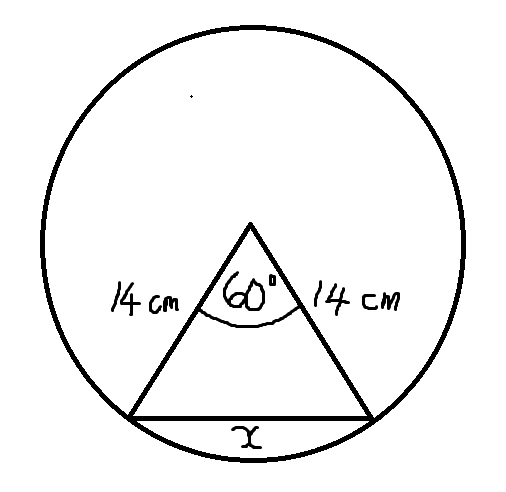$x^2 = 14^2 + 14^2 - 2(14)(14) \cos 60^\circ...Cosine\;\;Law \\[3ex] x^2 = 196 + 196 - 392\left(\dfrac{1}{2}\right) \\[5ex] x^2 = 392 - 196 \\[3ex] x^2 = 196 \\[3ex] x^2 = 196 \\[3ex] x = \sqrt{196} \\[3ex] x = 14\;cm$

Use the following information to answer questions $3 - 5$

ACT In the standard $(x, y)$ coordinate plane below, $\triangle AOB$ is formed by $\overleftrightarrow{AB}$, the $x-axis$, and the $y-axis$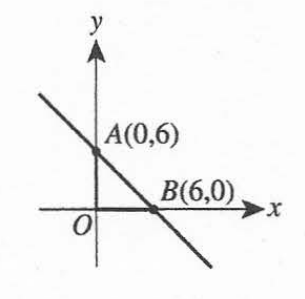(3.) ACT What is the area of $\triangle AOB$ in square coordinate units?

$A.\;\; 6 \\[3ex] B.\;\; 6\sqrt{2} \\[3ex] C.\;\; 12 \\[3ex] D.\;\; 18 \\[3ex] E.\;\; 36 \\[3ex]$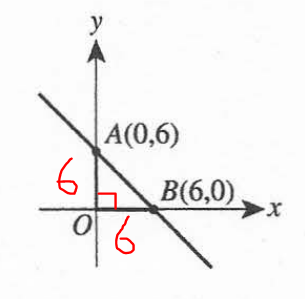$\underline{\triangle OAB} \\[3ex] Area = \dfrac{1}{2} * OA * OB * \sin \angle AOB \\[5ex] Area = \dfrac{1}{2} * 6 * 6 * \sin 90 \\[5ex] Area = 3 * 6 * 1 \\[3ex] Area = 18 \;\;square\;\;units$
(4.) ACT What is the length of $\overline{AB}$ in coordinate units?

$F.\;\; 2\sqrt{6} \\[3ex] G.\;\; 6\sqrt{2} \\[3ex] H.\;\; 6\sqrt{3} \\[3ex] J.\;\; 6 \\[3ex] K.\;\; 12 \\[3ex]$$\underline{\triangle OAB} \\[3ex] hyp^2 = leg^2 + leg^2 ... Pythagorean\;\;theorem \\[3ex] leg = 6 \\[3ex] leg = 6 \\[3ex] hyp^2 = 6^2 + 6^2 \\[3ex] hyp^2 = 36 + 36 \\[3ex] hyp^2 = 72 \\[3ex] hyp = \sqrt{72} \\[3ex] hyp = \sqrt{36 * 2} \\[3ex] hyp = \sqrt{36} * \sqrt{2} \\[3ex] hyp = 6 * \sqrt{2} \\[3ex] hyp = 6\sqrt{2}$
(5.) ACT Which of the following is an equation of $\overleftrightarrow{AB}$ ?

$A.\;\; y = -x + 6 \\[3ex] B.\;\; y = x - 6 \\[3ex] C.\;\; y = x + 6 \\[3ex] D.\;\; y = -6x - 6 \\[3ex] E.\;\; y = 6x + 6 \\[3ex]$

$A = Point\;\;1 = (0, 6) \\[3ex] x_1 = 0 \\[3ex] y_1 = 6 \\[3ex] B = Point\;\;2 = (6, 0) \\[3ex] x_2 = 6 \\[3ex] y_2 = 0 \\[3ex] Slope = m = \dfrac{y_2 - y_1}{x_2 - x_1} \\[5ex] m = \dfrac{0 - 6}{6 - 0} \\[5ex] m = \dfrac{-6}{6} \\[5ex] m = -1 \\[3ex] b = y-intercept = 6 \\[3ex] Equation:\;\; y = mx + b \\[3ex] y = -1 * x + 6 \\[3ex] y = -x + 6$
(6.) JAMB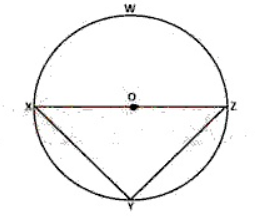In the diagram above, $\overline{XZ}$ is the diameter of the circle XYZW, with the centre O and radius $\dfrac{15}{2}\;cm$
If $|XY| = 12\;cm$, find the area of the triangle.

$A.\;\; 45\;cm^2 \\[3ex] B.\;\; 27\;cm^2 \\[3ex] C.\;\; 75\;cm^2 \\[3ex] D.\;\; 54\;cm^2 \\[3ex]$

$Radius = OX = OZ = \dfrac{15}{2} \\[5ex] Diameter = XZ = 2 * \dfrac{15}{2} = 15 \\[5ex] \underline{\triangle XYZ} \\[3ex] hyp^2 = leg^2 + leg^2 ...Pythagorean\;\;theorem \\[3ex] hyp = XZ = 15\;cm \\[3ex] leg = XY = 12\;cm \\[3ex] leg = YZ = ? \\[3ex] \implies \\[3ex] 15^2 = 12^2 + leg^2 \\[3ex] leg^2 = 15^2 - 12^2 \\[3ex] leg^2 = 225 - 144 \\[3ex] leg^2 = 81 \\[3ex] leg = \sqrt{81} \\[3ex] leg = 9\;cm \\[3ex] \underline{Circle\;XYZW} \\[3ex] \angle XYZ = 90^\circ...\angle \;\;in\;\;a\;\;semicircle \\[3ex] Area\;\;of\;\;\triangle XYZ \\[3ex] = \dfrac{1}{2} * XY * YZ * \sin \angle XYZ \\[5ex] = \dfrac{1}{2} * 12 * 9 * \sin 90 \\[5ex] = 6 * 9 * 1 \\[3ex] = 54\;cm^2$
(7.) CSEC (a)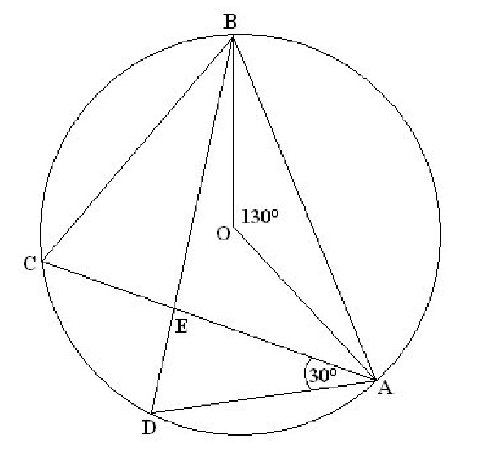In the diagram above, not drawn to scale, $O$ is the centre of the circle.
$\angle AOB = 130^\circ$, $\angle DAE = 30^\circ$, and $AEC$ and $BED$ are chords of the circle.
(a) Calculate the size of EACH of the following angles, giving reasons for EACH step of your answers.

$(i)\:\: \angle ACB \\[3ex] (ii)\:\: \angle CBD \\[3ex] (iii)\:\: \angle AED \\[3ex]$ (b) Show that $\triangle BCE$ and $\triangle ADE$ are similar.

(c) Given that CE = 6 cm, EA = 9.1 cm and DE = 5 cm,
(i) calculate the length of EB
(ii) calculate correct to 1 decimal place the area of $\triangle AED$

The (a) part is Circle Theorems

$(i) \\[3ex] \angle BOA = 2 * \angle ACB \\[3ex] ...\angle \:\:at\:\:center\:\:is\:\:twice\:\:\angle\:\:at\:\:circumference \\[3ex] 130 = 2 * \angle ACB \\[3ex] \angle ACB = \dfrac{130}{2} \\[5ex] \angle ACB = 65^\circ \\[3ex] (ii) \\[3ex] \angle CBD = \angle CAD = 30^\circ ...\angle s\:\:in\:\:same\:\:segment\;\;are\;\;equal \\[3ex] (iii) \\[3ex] \angle ADB = \angle ACB = 65^\circ ...\angle s\:\:in\:\:same\:\:segment\;\;are\;\;equal \\[3ex] \underline{\triangle AED} \\[3ex] \angle DAE + \angle AED + \angle EDA = 180 ...sum\:\:of\:\:\angle s\:\:of\:\:\triangle AED \\[3ex] \angle EDA = \angle ADB = 65 ...diagram \\[3ex] 30 + \angle AED + 65 = 180 \\[3ex] \angle AED + 95 = 180 \\[3ex] \angle AED = 180 - 95 \\[3ex] \angle AED = 85^\circ \\[3ex] (b) \\[3ex] \underline{\triangle BCE} \\[3ex] \angle BCE = \angle ACB = 65^\circ ...diagram \\[3ex] \angle CBE = \angle CBD = 30^\circ ...diagram \\[3ex] \underline{\triangle ADE} \\[3ex] \angle ADE = \angle BCE = 65^\circ ...\angle s\:\:in\:\:same\:\:segment\;\;are\;\;equal \\[3ex] \angle DAE = 30^\circ...given \\[3ex] Because: \\[3ex] \angle BCE = \angle ADE = 65^\circ \\[3ex] And: \\[3ex] \angle CBE = \angle DAE = 30^\circ \\[3ex] \therefore \triangle BCE \sim \triangle ADE ...AA\sim\;\;Postulate \\[3ex] (c) \\[3ex] (i) \\[3ex] \dfrac{|EB|}{|EA|} = \dfrac{|CE|}{|DE|} ...Scale\;\;Factors \\[5ex] \dfrac{|EB|}{9.1} = \dfrac{6}{5} \\[5ex] |EB| = \dfrac{9.1(6)}{5} \\[5ex] |EB| = \dfrac{54.6}{5} \\[5ex] |EB| = 10.92\;cm \\[3ex] (ii) \\[3ex] \underline{\triangle AED} \\[3ex] \angle AED + \angle ADE + \angle DAE = 180^\circ ...sum\;\;of\;\;\angle s\;\;of\;\;\triangle AED \\[3ex] \angle AED + 65 + 30 = 180 \\[3ex] \angle AED + 95 = 180 \\[3ex] \angle AED = 180 - 95 \\[3ex] \angle AED = 85^\circ \\[3ex] Area\;\;of\;\;\triangle AED \\[3ex] = \dfrac{1}{2} * |EA| * |DE| * \sin \angle AED \\[5ex] = 0.5 * 9.1 * 5 * \sin 85^\circ \\[5ex] = 22.75 * 0.9961946981 \\[3ex] = 22.66342938 \\[3ex] \approx 22.7\;cm^2$
(8.) ACT Graphed in the standard $(x, y)$ coordinate plane below is line l and the circle with equation $(x - 2)^2 + y^2 = 1$
Line l passes through $O(0, 0)$ and is tangent to the circle at A, and B is the center of the circle.
What is the measure of $\angle AOB$?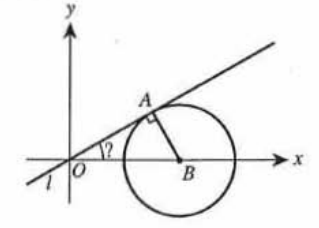$F.\;\; 15^\circ \\[3ex] G.\;\; 22.5^\circ \\[3ex] H.\;\; 30^\circ \\[3ex] J.\;\; 45^\circ \\[3ex] K.\;\; 60^\circ \\[3ex]$

$Based\;\;on\;\; (x - 2)^2 + y^2 = 1 \\[3ex] (x - 2)^2 + (y - 0)^2 = 1^2 \\[3ex] \implies \\[3ex] Circle\;\;center = (2, 0)...at\;\;B \\[3ex] \implies OB = 2 \\[3ex] Radius = AB = 1 \\[3ex]$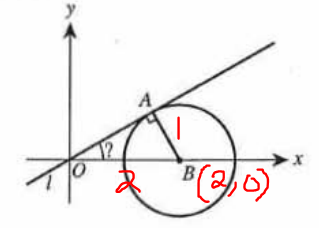$\underline{\triangle OAB} \\[3ex] \dfrac{\sin \angle AOB}{1} = \dfrac{\sin 90}{2} \\[5ex] \sin \angle AOB = \dfrac{1}{2} \\[5ex] \angle AOB = \sin^{-1}\left(\dfrac{1}{2}\right) \\[5ex] \angle AOB = 30^\circ$
(9.) NZQA C is the centre of the circle.
The radius of the circle is 28 mm.
Straight lines LMT and LNS are tangents to the circle at points M and N, respectively.
Angle MLN = 72°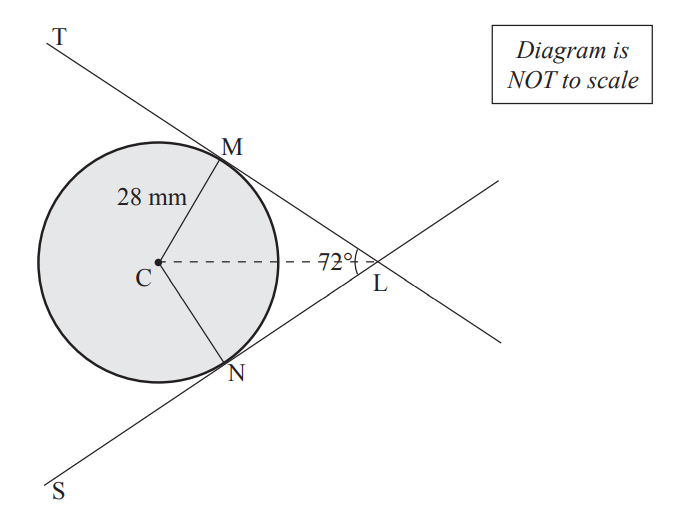Calculate the length LC

$\angle CML = 90^\circ ... radius\;CM \perp tangent\;LMT \;\;at\;\;point\;\;of\;\;contact\;M \\[3ex] \angle CLM = \dfrac{1}{2} * 72 \\[3ex] ... the\;\;line\;CL\;\;joining\;\;the\;\;external\;\;point\;\;from\;\;two\;\;tangents\;\;and\;\;the\;\;centre\;C\;\;bisects\;\;the\;\;\angle\;\;between\;\;the\;\;tangents \\[3ex] \angle CLM = 36^\circ \\[3ex]$$\underline{\triangle CLM} \\[3ex] \sin \angle CLM = \dfrac{|MC|}{|LC|} ... SOHCAHTOA \\[5ex] \sin 36 = \dfrac{28}{|LC|} \\[5ex] |LC| * \sin 36 = 28 \\[3ex] |LC| = \dfrac{28}{\sin 36} \\[5ex] |LC| = \dfrac{28}{0.5877852523} \\[5ex] |LC| = 47.63644527\;mm$
(10.) CSEC Given that $\sin\theta = \dfrac{\sqrt{3}}{2},\;\;\; 0^\circ \le \theta \le 90^\circ$

(i.) Express in fractional or surd form the value of $\cos\theta$

(ii.) Show that the area of triangle $CDE$ is $150\sqrt{3}$ square units, where $CD = 30$ units and $DE = 20$ units.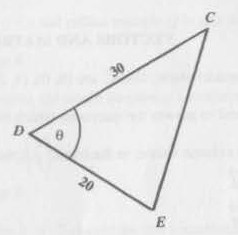(iii.) Calculate the length of the side $EC$

$(i.) \\[3ex] 0^\circ \le \theta \le 90^\circ \\[3ex] SOH:CAH:TOA \\[3ex] \sin \theta = \dfrac{\sqrt{3}}{2} = \dfrac{opp}{hyp} \\[5ex] opp = \sqrt{3} \\[3ex] hyp = 2 \\[3ex] hyp^2 = opp^2 + adj^2 ...Pythagorean\;\;theorem \\[3ex] 2^2 = (\sqrt{3})^2 + adj^2 \\[3ex] 4 = 3 + adj^2 \\[3ex] 4 - 3 = adj^2 \\[3ex] 1 = adj^2 \\[3ex] adj^2 = 1 \\[3ex] adj = \sqrt{1} \\[3ex] adj = 1 \\[3ex] \cos \theta \\[3ex] = \dfrac{adj}{hyp} \\[5ex] = \dfrac{1}{2} \\[5ex] (ii.) \\[3ex] \underline{\triangle CDE} \\[3ex] \cos \theta = \dfrac{1}{2} \\[5ex] \theta = \cos^{-1}\left(\dfrac{1}{2}\right) \\[5ex] \theta = 60^\circ \\[3ex] Area = \dfrac{1}{2} * |CD| * |DE| * \sin\theta \\[5ex] = \dfrac{1}{2} * 30 * 20 * \sin 60 \\[5ex] = 15 * 20 * \dfrac{\sqrt{3}}{2} \\[5ex] = 15 * 10 * \sqrt{3} \\[3ex] = 150\sqrt{3}\;square\;\;units \\[3ex] (iii.) \\[3ex] |EC|^2 = |CD|^2 + |DE|^2 - 2(|CD|)(|DE|)\cos\theta...Cosine\;\;Law \\[3ex] |EC|^2 = 30^2 + 20^2 - 2(30)(20) * \cos 60 \\[3ex] |EC|^2 = 900 + 400 - 1200 * \dfrac{1}{2} \\[5ex] |EC|^2 = 1300 - 600 \\[3ex] |EC|^2 = 700 \\[3ex] |EC| = \sqrt{700} \\[3ex] |EC| = 26.45751311 \\[3ex] |EC| \approx 26\;units$
(11.) curriculum.gov.mtPRT is a triangle with points Q and S on PR and RT respectively such that SQ is parallel to TP.

(a) Show that triangles RTP and RSQ are similar.

(b) Calculate the size of SQ

(c) Given that the area of triangle RTP is 35 $cm^2$, calculate:
(i) the area of triangle RSQ
(ii) the area of quadrilateral QSTP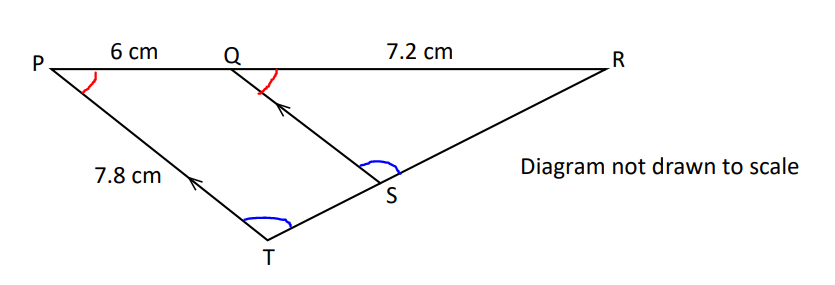$(a) \\[3ex] \angle RQS = \angle RPT...corresponding\;\;\angle s\;\;are\;\;equal \\[3ex] \angle RSQ = \angle RTP ...corresponding\;\;\angle s\;\;are\;\;equal \\[3ex] \angle QRS = \angle PRT...common\;\;\angle\;\;R \\[3ex] Any\;\;of\;\;the\;\;two\;\;can\;\;be\;\;used\;\;for\;\;the\;\;AA\sim\;\;Postulate \\[3ex] \therefore \triangle RTP \sim \triangle RSQ...AA\sim\;\;Postulate \\[3ex] (b) \\[3ex] \dfrac{|SQ|}{7.8} = \dfrac{7.2}{7.2 + 6} \\[5ex] \dfrac{|SQ|}{7.8} = \dfrac{7.2}{13.2} \\[5ex] |SQ| = \dfrac{7.8(7.2)}{13.2} \\[5ex] |SQ| = \dfrac{56.16}{13.2} \\[5ex] |SQ| = 4.254545455 \\[3ex] |SQ| \approx 4.3\;cm \\[3ex] (c) \\[3ex] (i) \\[3ex] Scale\;\;Factor = \dfrac{|RQ|}{|RP|} \\[5ex] Area\;\;Ratio = \dfrac{|RQ|^2}{|RP|^2} ...Area\;\;Ratio-Scale\;\;Factor\;\;Theorem \\[5ex] Also: \\[3ex] Area\;\;Ratio = \dfrac{Area\;\;of\;\;\triangle RSQ}{Area\;\;of\;\;\triangle RTP} \\[5ex] \implies \\[3ex] \dfrac{Area\;\;of\;\;\triangle RSQ}{Area\;\;of\;\;\triangle RTP} = \dfrac{|RQ|^2}{|RP|^2} \\[5ex] \dfrac{Area\;\;of\;\;\triangle RSQ}{35} = \dfrac{(7.2)^2}{(7.2 + 6)^2} \\[5ex] \dfrac{Area\;\;of\;\;\triangle RSQ}{35} = \dfrac{(7.2)^2}{(13.2)^2} \\[5ex] \dfrac{Area\;\;of\;\;\triangle RSQ}{35} = \dfrac{51.84}{174.24} \\[5ex] Area\;\;of\;\;\triangle RSQ \\[3ex] = \dfrac{35 * 51.84}{174.24} \\[5ex] = \dfrac{1814.4}{174.24} \\[5ex] = 10.41322314 \\[3ex] \approx 10.4\;cm^2 \\[3ex] (ii) \\[3ex] Area\;\;of\;\;Quadrilateral\;QSTP = Area\;\;of\;\;\triangle RTP - Area\;\;of\;\;\triangle RSQ ...diagram \\[3ex] = 35 - 10.41322314 \\[3ex] = 24.58677686 \\[3ex] \approx 24.6\;cm^2$
(12.) CSEC The digram below, not drawn to scale, shows the sector of a circle with centre $O$
$\angle MON = 45^\circ$ and $ON = 15\;cm$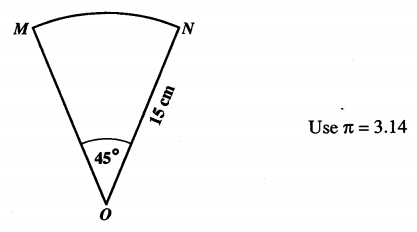(i.) the length of the minor arc MN
(ii.) the perimeter of the figure MON
(iii.) the area of the figure MON

$(i.) \\[3ex] Length\;\;of\;\;arc\;MN = L \\[3ex] L = \dfrac{2\pi r\theta}{360} ...\theta \:\:in\:\:DEG \\[5ex] \pi = 3.14 \\[3ex] \theta = 45^\circ \\[3ex] r = 15\;cm \\[3ex] L = \dfrac{2 * 3.14 * 15 * 45}{360} \\[5ex] L = \dfrac{4239}{360} \\[5ex] L = 11.775 \\[3ex] L \approx 11.78\;cm \\[3ex] (ii.) \\[3ex] \underline{Figure\;\;MON} \\[3ex] Perimeter = r + r + L \\[3ex] Perimeter = 15 + 15 + 11.775 \\[3ex] Perimeter = 41.775 \\[3ex] Perimeter \approx 41.78\;cm \\[3ex] (iii.) \\[3ex] \underline{Figure\;\;MON} \\[3ex] Area\;\; of\;\; Figure\;\;MON = Area\;\;of\;\;sector\;OMN = A \\[3ex] A = \dfrac{\pi r^2\theta}{360} ...\theta \:\:in\:\:DEG \\[5ex] A = \dfrac{3.14 * 15^2 * 45}{360} \\[5ex] A = \dfrac{31792.5}{360} \\[5ex] A = 88.3125 \\[3ex] A \approx 88.31\;cm^2$
(13.)

(14.)


(15.)

(16.)